# Logic Gates- Type of Logic Gate, Number System

A logic gate is a digital circuit which is based on certain logical relationship between the input and the output voltages of the circuit.

1. The logic gates are built using the semiconductor diodes and transistors.
Each logic gate is represented by its characteristic symbol.
2. The operation of a logic gate is indicated in a table, known as truth table. This table contains all possible combinations of inputs and the corresponding outputs.

3. A logic gate is also represented by a Boolean algebraic expression. Boolean algebra is a method of writing logical equations showing how an output depends upon the combination of inputs. Boolean algebra was invented by George Boole.

TYPES OF LOGIC GATES:

OR Gate :

The out of an OR gate will be one if at least value of one input  is 1.

Logic Symbol of OR Gate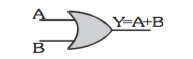The Boolean expression of OR gate is Y = A + B, read as Y equals A 'OR' B.

Truth table representation of Two input OR Gate.

 A B Y 0 0 0 1 0 1 0 1 1 1 1 1

AND gate :

The output of AND will be 1 if the value of all input is 1.

Logical Symbol of AND Gate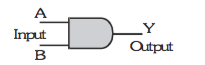The Boolean expression of AND gate is Y = A.B

Truth table for AND Gate

 A B Y 1 1 1 1 0 0 0 1 0 0 0 1

Not Gate :

The output of a NOT gate attains the state 1 if and only if the input does not attain the state 1.

Logical Symbol of NOT gate.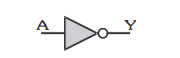Truth Table of Not Gate

 A Y 1 0 0 1

COMBINATION OF GATES :

The three basis gates (OR, AND and NOT) when connected in various combinations give us logic gates such as NAND, NOR gates, which are the universal building blocks of digital circuits .

NAND Gate :

Logical Symbol of NAND Gate: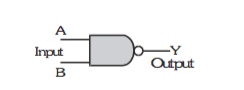Truth Table of NAND Gate:

 A B Y 0 0 1 0 1 1 1 0 1 1 1 0

NOR Gate :

Logical Symbol of NOR Gate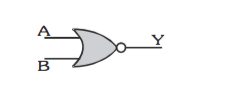Truth table of NOR Gate:

 A B Y 0 0 1 0 1 0 1 0 0 1 1 0

XOR AND XNOR GATES

The Exclusive - OR gate (XOR gate)

The output of a two-input XOR gate attains the state 1 if one and only one input attains the state 1.

Logical Symbol of XOR Gate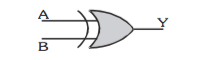Truth table of XOR Gate

 A B Y 0 0 0 0 1 1 1 0 1 1 1 0

Exclusive - NOR gate (XNOR gate)

The output is in state 1 when its both inputs are the same that is, both 0 or both 1.

Logical Symbol :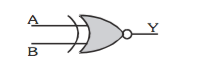Truth Table

 A B Y 0 0 1 0 1 0 1 0 0 1 1 1

LAWS OF BOOLEAN ALGEBRA:

Basic OR, AND, and NOT operations are given below

 OR AND NOT A+0 = A A.0 = 1 A + A` = 1 A+1 = 1 A.1 = A A.A` = 0 A+A = A A.A = A A``.A = A

Boolean algebra obeys commutative, associative and distributive laws as given below :

Commutative laws :

A+B=B+A;

A.B = B.A

Associative laws :

A + (B + C) = (A + B) + C

A. (B . C) = (A. B) . C

Distributive laws :

A. (B + C) = A.B + A.C

Some other useful identities :

(i) A + AB = A
(iii) A.(A+ B)=A
(v) A+(B.C)=(A+B).(A+C)

De Morgan's theorem :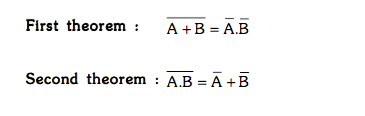NUMBER SYSTEMS

Decimal Number system

The base of this system is 10 and in this system 10 numbers [0,1,2,3,4,5,6,7,8,9] are used.

Ex. 1396, 210.75 are decimal numbers.

Binary Number System

The base of this system is 2 and in this system 2 numbers (0 and 1) are used.

Ex. 1001, 1101.011 are Binary numbers.

Binary to decimal conversion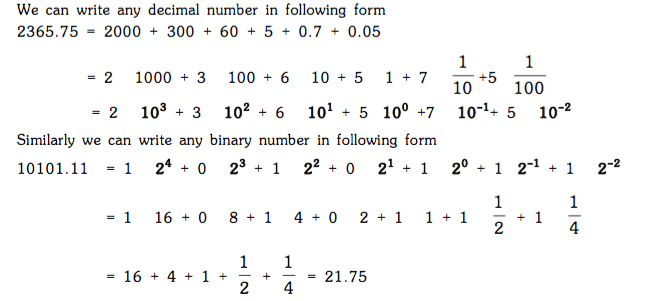Example :

Convert binary number 1011.01 into decimal number.

Solution :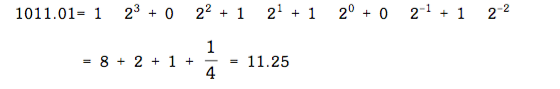Example :

Convert binary number 1000101.101 into decimal number.

Solution :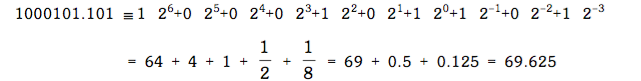Post By : Amit Rai 23 Apr, 2019 3442 views Physics